# CHEMICAL BONDING

## What is Chemical Bonding?

A chemical bond is a lifelong attraction for chemical compounds containing atoms, ions, or molecules.

The relation can be created by the electrostatic force of pull between oppositely charged ions such as in ionic bonds or by the electron sharing as in covalent connections.

## Ionic Bond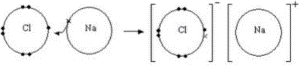ntent -->

The ionic bond is the electrostatic attracting force between opposing ions produced by the direct movement of electrons from an atom to another. An ionic bond is also called an electrovalent bond

• A positive ion(cation) forms an atom (usually a metal) which loses an electron(s).

• The electron is passed to a separate atom. A negative ion(anions) is formed by the atom where the electron (usually not a metal) absorbs.

• Strong electrostatic attraction attracts cations and anions. The attractive influence is the ionic relation

• The attraction force between cation and anion is very strong, and it’s a strong ionic relation.

• The non-directional ionic bonds will attract each cation and each adjacent anion will form a large ionic grid. The ionic binding compound is called sodium chloride an example, NaCl.

• Dot-and-cross diagram

• the outer electron shell only.

• that the charge of the ion is spread evenly using a square bracket.

• the charge of each ion.

It is also called the Lewis diagram

Using a dot-and-cross diagram to represent the formation of ionic bond: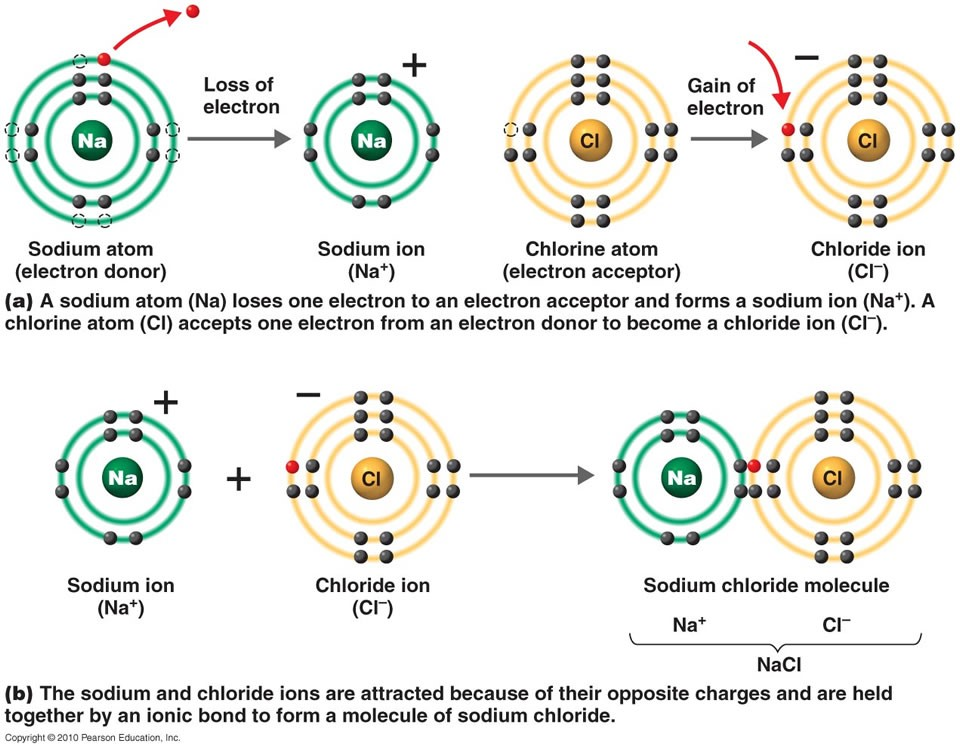Strength of ionic bonds

• The strength of the ionic bond is a measure of the electrostatic force of attraction between the ions

• The force of attraction between the oppositely-charged ions is proportional to the charge on the ions and inversely proportional to the square of the distance between the ions.

• The strength of the ionic bond is manifested in the melting point of the ionic compound.
• For instance, the melting point of NaCl is higher than NaBr

• This is because the size of Br⁻ ion is larger than Cl⁻ ion. Therefore, the distance between Br⁻ and Na⁺ is larger than that between Cl⁻ and Na⁺.

• As a result, the electrostatic force of attraction between Na⁺ and Cl⁻ is stronger than that of between Na⁺ and Br⁻ ion.
• The melting point of NaCl is lower than MgCl2.

• This is because Mg²⁺ ion has a higher charge than Na⁺ Besides that, the size of Mg²⁺ ion is smaller than Na⁺ ion.

• The above two factors cause the electrostatic force of attraction between Mg²⁺ and Cl⁻ to be stronger than that of between Na⁺ and Cl⁻.

## COVALENT BONDING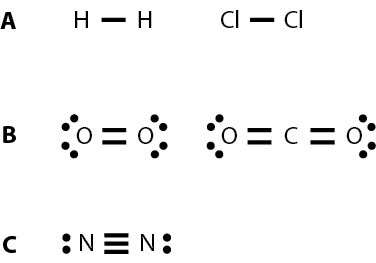A covalent bond is a chemical bond that comprises exchanging electron pairs among atoms, also known as the molecular bond. Such pairs of electrons are called mutual pairs or pairs, and a constant balancing of attractive and repulsive forces between atoms is known as covalent interaction as they exchange electrons.

## Single bond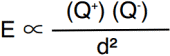A single bond is made when two atoms share a pair of electrons.

Examples of compounds with single bonds:

## Double bond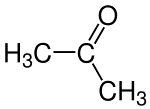A double bond is formed when two pairs of electrons are shared between two atoms.

Examples of compounds with double bonds:

## Triple bond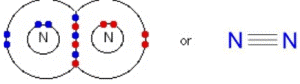A triple bond is formed when three pairs of electrons are shared between two atoms.

Examples of compounds with triple bonds:

Lone pair and bond pair of electrons

1) The pair of electrons used in covalent bonding is called the bond pair while the pair of electrons not used in covalent bonding is called the lone pair.

Octet-deficient and expanded octet species

Atoms typically break up the electrons to a duplicate/octet structure – the byte law

• The main atom contains fewer than eight electrons in octet-deficient animals.

• Sources of this include trifluoride boron, BF3, and monoxide nitrogen NO.

• The central atom has more than eight electrons in extended byte material.

• One source is chloride phosphorus(V), PCL

• It is only possible for three elements and more, as atoms have empty d orbits to hold more than eight electrons from period 3.

## Valence shell electron pair repulsion(VSEPR) theory: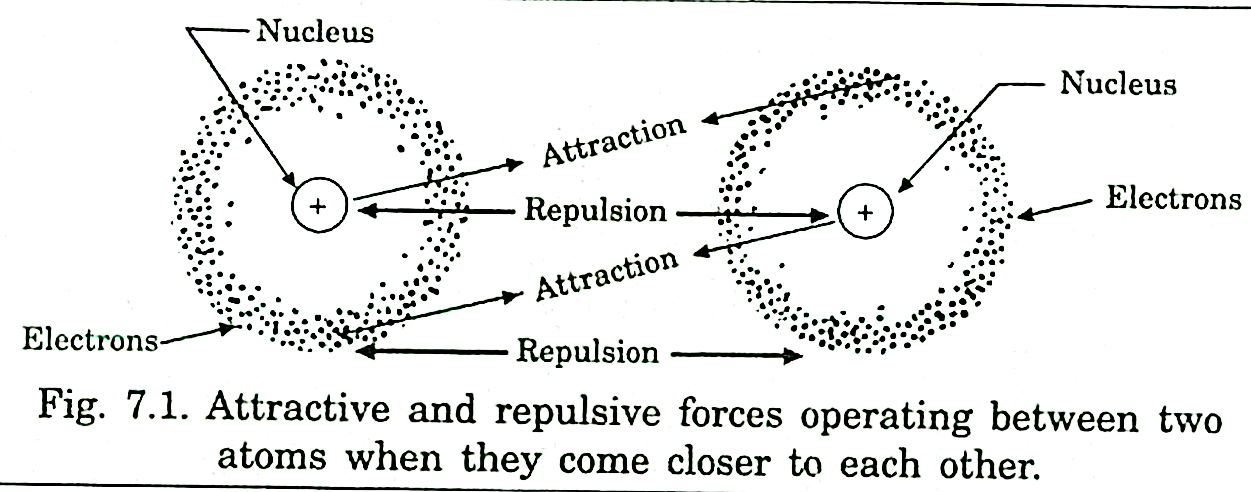All electrons are negatively-charged, so they will repel each other when they are close together

So, a pair of electrons in the bonds surrounding the central atom in a molecule will repel the other electron pairs. The amount of repulsion is as follow:

General steps to determine the shape of a molecule:

determine the number of valence electrons in the central atom

find the total number of electrons surrounding the central atom by adding the number of shared electrons to it. (Dot-and-cross diagram might be necessary)

find the number of electron pairs by dividing the total number of electrons by two

determine how many pairs is/are bond pairs and lone pairs. (A double bond or triple bond is counted as one bond pair)

refer to the table to obtain the shape of the molecule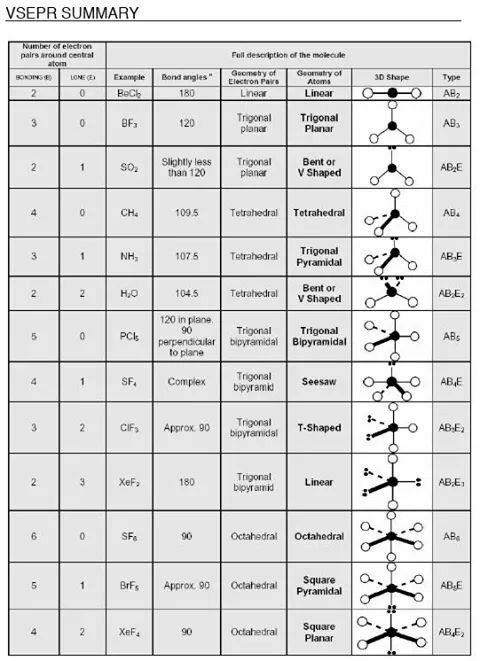Effect of  lone pair on the bond angle

For methane, ammonia, and water, the electron pair geometries are tetrahedral. However, the molecular geometries are different

In methane, all the bonds are identical, the repulsion between the bonds is the same. Thus, methane has a perfect tetrahedral structure with a bond angle of 109.5°.

In ammonia, the repulsion between the lone pair and the bond pairs is stronger than in This forces the bond angle to decrease slightly to 107°.

In water, there are two lone pairs and thus the repulsion is the greatest, the two bond pairs are pushed closer to one another and the bond angle is reduced to 104.5°.

## Effect of electronegativity on the bond angle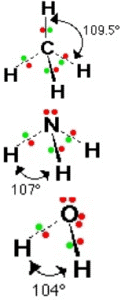Water and hydrogen sulfide has the same general shape with the same number of bond pairs and lone pairs. However, their bond angles are different

This is because oxygen has a higher electronegativity than The bond pairs of electrons are closer to the oxygen atom compared to the sulfur atom.

This results in greater repulsion in the O-H bonds than in the S-H bonds. Therefore, the bond angle increases from 92.5° to 104.5°.

## Sigma(σ) bond and pi(π) bond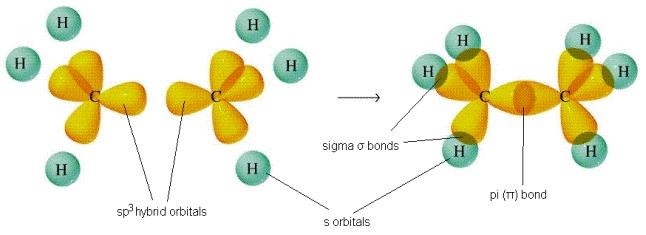A sigma bond is formed by orbitals from two atoms overlapping end-to-end.

In a sigma bond, the electron density is concentrated between the two nuclei

A pi bond is formed by the p orbitals from two atoms overlapping sideways.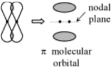In a pi bond, there are two regions of high electron density alongside the nuclei.

A pi bond is weaker than a sigma bond because the overlapping of charge clouds is less than in a sigma bond

In covalent molecules, single bonds are sigma bonds(σ), a double bond consists of one sigma bond and one pi bond(1σ, 1π), and a triple bond consists of one sigma bond and two pi bonds(1σ, 2π).

#### Related Posts